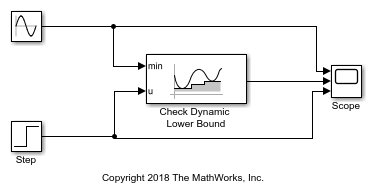# Check Signal Lower Bound with Check Dynamic Lower Bound Block

Using the Check Dynamic Lower Bound block, you can check if an input signal falls below a changing lower bound during a simulation.In this example, the Check Dynamic Lower Bound block compares the value of a tested input signal from a Step block at the u port to a Sine Wave block at the min port. The Check Dynamic Lower Bound block checks if the value of the signal at the min port is less than the value of the tested input sigal. If it is, the block asserts `true (1)`. Because the Output assertion signal parameter of the Check Dynamic Lower Bound block is selected, the block outputs the assertion value. Run the simulation to observe the model output.At the start of the simulation, the Check Dynamic Lower Bound block outputs `0` because the value of the Sine Wave block is greater than the value of the Step block. At a time of `3.14`, the sine wave dips below `0`, causing the value of the Step block to exceed the sine wave. The Check Dynamic Lower Bound block recognizes this change and outputs `1`.

At a time of `5`, the Step block outputs a value of `0.5`, which is still greater than the sine wave. The Step block value stays greater than the sine wave until the time reaches `6.81`. The Check Dynamic Lower Bound block recognizes this change and the assertion fails. The assertion stays at `0` until the time reaches `8.90`, where the sine wave goes below the value of the Step block.